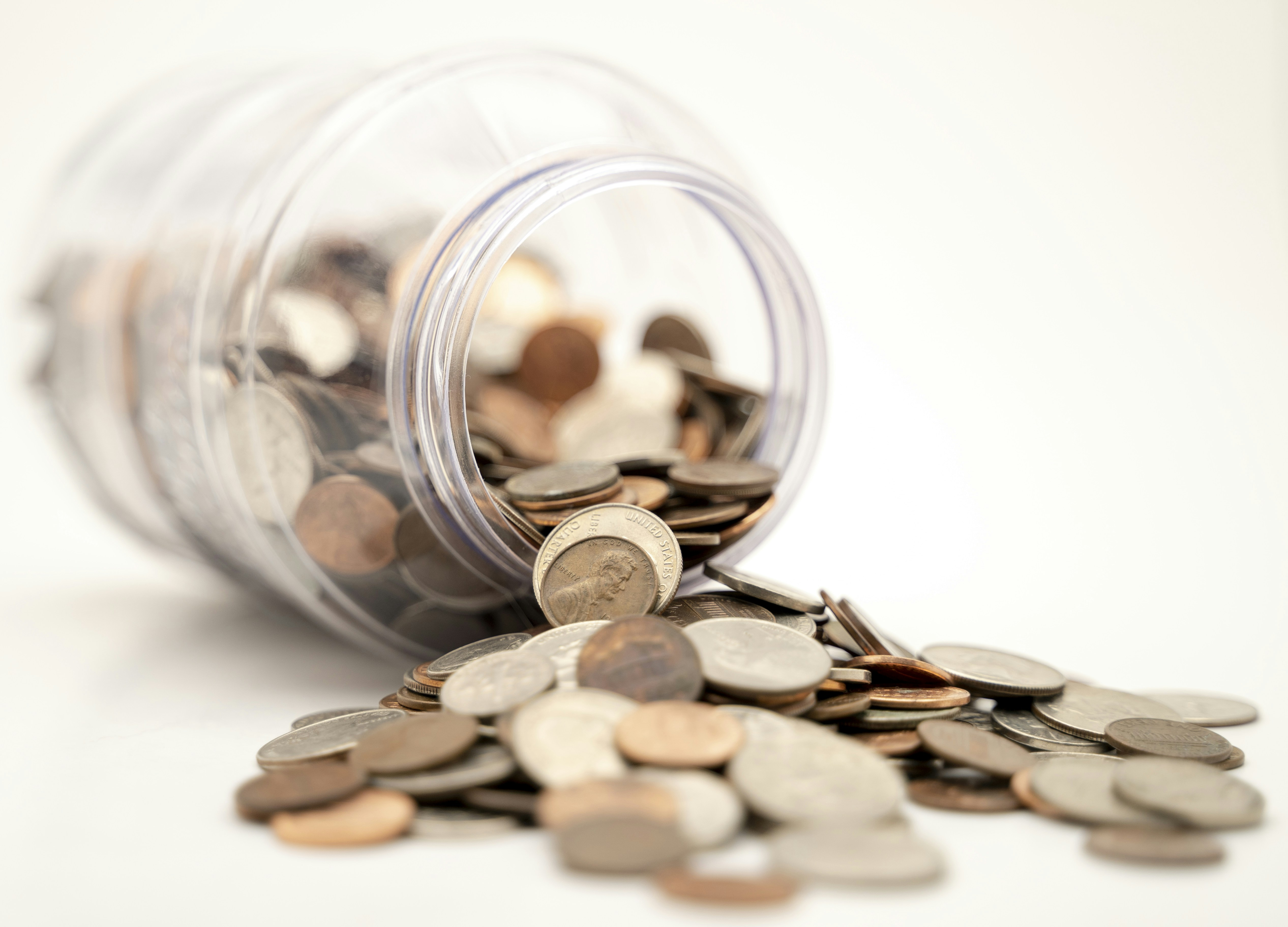Hackerrank - The Coin Change Problem Solution

# Hackerrank - The Coin Change Problem SolutionYou are working at the cash counter at a fun-fair, and you have different types of coins available to you in infinite quantities. The value of each coin is already given. Can you determine the number of ways of making change for a particular number of units using the given types of coins?

For example, if you have  types of coins, and the value of each type is given as  respectively, you can make change for  units in three ways: , , and .

Function Description

Complete the getWays function in the editor below. It must return an integer denoting the number of ways to make change.

getWays has the following parameter(s):

• n: an integer, the amount to make change for
• c: an array of integers representing available denominations

Input Format

The first line contains two space-separated integers describing the respective values of  and , where:
is the number of units
is the number of coin types
The second line contains  space-separated integers describing the respective values of each coin type :  (the list of distinct coins available in infinite amounts).

Constraints

• Each  is guaranteed to be distinct.

Hints

Solve overlapping subproblems using Dynamic Programming (DP):
You can solve this problem recursively but will not pass all the test cases without optimizing to eliminate the overlapping subproblems. Think of a way to store and reference previously computed solutions to avoid solving the same subproblem multiple times. * Consider the degenerate cases:
- How many ways can you make change for  cents? - How many ways can you make change for  cents if you have no coins? * If you're having trouble defining your solutions store, then think about it in terms of the base case . - The answer may be larger than a -bit integer.

Output Format

Print a long integer denoting the number of ways we can get a sum of  from the given infinite supply of  types of coins.

Sample Input 0

4 3
1 2 3

Sample Output 0

4

Explanation 0

There are four ways to make change for  using coins with values given by :

Thus, we print  as our answer.

Sample Input 1

10 4
2 5 3 6

Sample Output 1

5

Explanation 1

There are five ways to make change for  units using coins with values given by :

Thus, we print  as our answer.

### Solution in Python

def getWays(n, c):
n_perms = +*n
for coin in c:
for i in range(coin, n+1):
n_perms[i] += n_perms[i-coin]
return n_perms[n]

n, m = map(int, input().split())
c = list(map(int, input().split()))
print(getWays(n, c))